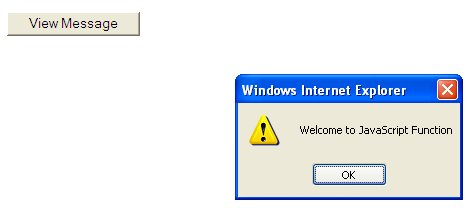# JavaScript Lesson 13: Introduction to Javascript Function

### 13.1 Definition of a Function

What is a function in JavaScript? A JavaScript function is a type of program that can be called from the main JavaScript program to perform certain tasks. We can also define a function as a module of the main JavaScript program. A professionally written JavaScript program usually consists of numerous functions. It is easier to construct, debug and maintain a large program that makes up of smaller modules, because the programmer needs only debug a module or modules if certain errors occur, instead of having to search for the whole program and trying to locate the error code.

There are two kinds of functions in JavaScript, the built-in functions, and the user-define functions. Built-in functions are also known as methods that are tied to certain objects. For example, functions that are tied to the Math object are round, pow and more. There are also string manipulation functions, date and time functions and many more,  please refer to our previous lessons for more information on these functions.

### 13.2 Creating User-Defined Functions

To create a JavaScript function, You can declare the variables by listing them within parentheses after the function name. These variables are also known as parameters. They are also known as the local variable, which means that they are known only in the function in which they are defined. When we pass values to the parameters, they are called arguments. After creating a function, you can call the function by using its name followed by the parentheses and the arguments within the parentheses.

The syntax of a function is:

```function   NameOfFuntion(parameter1, parameter2, parameter3,......)

{Statements}
```

For example, you can write a simple welcoming message function as follows:

```function Message()

{ alert("Welcome to JavaScript Function")
```

However, you need to create an event to call the function. Here I will show you how to create a click event. You can use the code as follow:

```<form>
<input type="button" onclick="Message()" value="View Message">
</form>
```

The complete program is shown in Example 13.1

#### Example 13.1

```<html>
<meta http-equiv="Content-Type" content="text/html; charset=windows-1252">
<title>Example 13.1</title>
<body>
<script language="javascript">

function Message()
{ alert("Welcome to JavaScript Function"); }

</script>
<form>
<input type="button" onclick="Message()" value="View Message">
</form>

</body>

</html>
```

The output is shown in the figure below. When you click the view message button, the Welcome to JavaScript Function message will pop up.Click on Example 13.1 to test the program

Let us look at another example. This example illustrates a financial calculator that calculates the future value of a present sum invested for a number of years compounded on a certain annual interest rate. In this program, we create a function that can be called to perform the calculation. This function comprises three parameters: Present value, the number of years and annual interest rate.

Let us use the function name FV to denote Future Value and use the parameters PV to denote present value, n to denote the number of years and r to denotes annual interest rate in percentage form. The formula to calculate the future value is

FV=PV(1+r/100)n

The function written in JavaScript is shown below

```function FV(PV, n, r)
{ FV=PV*Math.pow(1+r/100, n):
```

The fully functional future value calculator is shown in Example 13.2 below:

### Example 13.2

```<html>
<meta http-equiv="Content-Type" content="text/html; charset=windows-1252">
<title>Example 13.2</title>
<body>
<script>
PV=window.prompt( "Enter the present value:", "0" );
n=window.prompt( "Enter number of years :", "0" );
r=window.prompt( "Enter the annual rate:", "0" );

function FV(PV, n, r)
{ FV=PV*Math.pow(1+r/100, n);

document.write("The future value after "+n+" years is \$"+FV); }

</script>
<form>
<input value="Calculate">
</form>

</body>

</html>
```

When you load the web page, you will be prompted to enter the present value, the number of years and the annual interest rate. On clicking the calculate button, the output should be something like the following:

The future value of the principal sum of \$30000 after 20 years at an annual interest rate of 5% is \$79598.93115433266

Click on Example 13.2 to test the program.